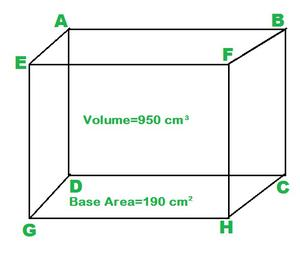Find the height of the cuboid whose base area is 180 cm2 and volume is 900 cm3

• Last Updated : 19 Oct, 2021

Mensuration is a subject of geometry that deals with geometric figure calculations like volume, surface area, lateral surface area, etc.

Cuboid is a solid bounded by six rectangular faces. It is a 3-dimensional shape with length, width, and height denoted by l,b, and h respectively. If you look around your room you can easily spot many cuboidal objects. The space occupied by a solid body is known as its volume. It is in cubic units. We can consider any face of a cuboid as its base then face above the base will be its top and four faces which are adjacent to the base and the top will be its lateral faces.

Total surface area of a cuboid is the sum of the area of all its faces (That is the area covered by all its faces). We know that the dimensions of opposite faces are the same therefore we multiply the area of three faces by 2. The unit of the surface area of a cuboid is given as unit2. Lateral surface area is the area covered by lateral faces.

Cuboid have two types of diagonals face diagonals and space diagonals. All the angles formed at the vertices of a cuboid are right angles and opposite faces of the cuboid are parallel to each otherCuboid

Consider the cuboid from the above figure it has:

(i) 6 faces: ABEF, ADGE, DCHG, BCHF, ABCD, EFGH.

(ii) 12 edges: AE, AD, AB, EG, EF, GD, GH, HC, HF, CB, CD, BF.

(iii) 8 vertices: A, B, C, D, E, F, G, H.

Formulas

Some important formulae are:

(i) Volume: l×b×h;

(ii) Total Surface Area (TSA): 2×(l×b+b×h+h×l)

(iii) Lateral Surface Area (LSA): 2×h×(l+b)

(iv) Diagonal: square root of (l×l+b×b+h×h)

Find the height of the cuboid whose base area is 180 cm2 and volume is 900 cm3.

Solution:

Given: Volume = 900 cm3, Base Area = 180 cm2.

Volume of a cuboid is: length × breadth × height (l×b×h).

Base area of cuboid is: length × breadth (l×b).

Volume = l×b×h = 900 cm3  ———–>1

Area = l×b = 180 cm2————->2

Substituting the value of l×b from equation 2 we get:

900 cm3 = 180 cm2 × h.

=> h = 900/180 cm

h = 5 cm.

Sample Problems

Question 1. Find the lateral surface area of a rectangular box whose height is 20 cm and the perimeter of its base is 250 cm.

Solution:

Given: perimeter of base = 250 cm

height h = 20 cm

we know that,

perimeter of base = 2×(l+b)

and lateral surface area lsa = 2×h×(l+b)

lsa = 2×(l+b)×h = 250×20 = 5000cm2.

Question 2. Find the amount of sand a box can hold whose length is 14cm, width is 8 cm and height is 12 cm.

Solution:

To know the amount of sand a box can hold we need to find its volume.

Volume = l×h×b

= 14×8×12

= 1344 cm3

Question 3. Find the sum of surface area covered by the base and the top face of a cuboid whose base area is 200 cm2.

Solution

We know that the opposite faces of a cuboid have the same dimensions. Hence the sum of surface area of base and top face will be:

2×BA = 2×200

= 400 cm2.

Question 4. Find the width of a cuboid whose volume is 300 cm3, length is 30 cm and height is 2 cm.

Solution:

Volume, V = l×b×h = 300

l = 30 cm

h = 2 cm

b = V/(l×h)

b = 300/(30×2)

= 10/2

= 5 cm

Question 5. Find the base area of a cuboid whose width is 20 cm and length is twice its width.

Solution:

Given:

b = 20 cm

l = 2×b = 2×20 = 40 cm

BA = l×b = 40×20

= 800 cm2.

My Personal Notes arrow_drop_up